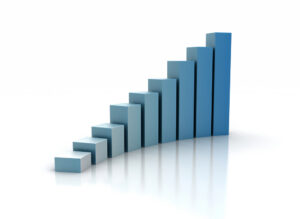Most people prefer to stay away from business finance, considering it difficult to understand. In reality, financial terms and concepts are not hard if they are approached in the right way. Moreover, in today’s world where everything can be learned over internet, why should you fear some financial terms? Today, let’s tackle the CAGR. If you go through financial journals or read performance reports of businesses you will definitely come across this term. Let us try to understand what CAGR is and how to calculate it. Trust me, I will keep things simple and not bore you with numbers.

## What is CAGR?

CAGR stands for Compound Annual Growth Rate. Did it ring any bells? Not yet. Don’t worry. Let me explain it first.

CAGR is not used as an accounting term. Rather, it is used to describe some specific elements like revenues or number of units delivered. It actually shows how a particular aspect of business has grown over time. So, when we are talking about revenue, it will tell you how revenue has grown over a specific time period.  Let us delve deeper and find out how it is used and calculated.

## How is CAGR used?

Returns from an investment varies over time. At times they go very high and may dip at some point also. The returns can be volatile and the returns can be withdrawn at different times too. In such conditions, it becomes impossible to compare between two investments and find out which one performed better. CAGR is used in such conditions to ease out the volatility. CAGR is actually the annual rate of return on an investment assuming that it has grown at the same rate over the period.

This is very useful if you want to calculate the returns on your mutual funds. The return mentioned for one, three or five year on fact sheets of mutual funds is CAGR.

## How is CAGR calculated?

To calculate CAGR you require certain data like the time period over which it is calculated and the initial value of investment and the final value of investment.

Here is the formula for CAGRLet me explain the symbols and then rewrite the formula for you.

t(0) = the starting period or the first year of investment

t(n) = the last year or the final year of investment

V(t0) = the initial investment or the start value

V(tn) = the final investment or the final value

The formula therefore states that

CAGR( from first year, to last year)The best thing about this formula is that it can be easily applied in excel. Only thing is that you have to apply the basics of mathematics that you learnt in your junior school and make sure that you consider the brackets carefully. Misplacing them will give you erroneous results.

Let us take an example and calculate the CAGR.

This is a revenue report for  marketing company for the period 2001-2004.

 year end 2001 2002 2003 2004 year-end revenue 9000 9550 11020 13000

Here,

t(n) – t(0) = 2004-2001 = 3

So,Let us take another example and calculate the CAGR, but this time in Excel with the help of predefined formula.In the above example,

The investment in \$ has been considered for 5 periods.

The formula for CAGR in Excel in known as XIRR

The syntax for the formula is

`XIRR (values, period)`

For our example

The syntax would change toThe XIRR calculated as per the record is 37.34%.

The figure of 37.345 signifies that over the period, the investment has yielded 37.34% return if we ignore the volatility of the returns. So, this is a smoothened value over the period concerned.

## Applications of CAGR

CAGR finds wide application in the business and financial sector. If there are two investments and the investors are interested to know which one is better in terms of return, they cannot rely on the direct revenue figures only. This is because, in terms of revenue figures, one may seem more lucrative but you also have to consider the amount of investment that has gone into it. In such cases, the investors rely on CAGR to ease out the volatility and calculate the rate of return.

While calculating the CAGRs of different investments, the period has to remain the same.

CAGR is also used to compare the historical returns of stocks or bonds.

## Other uses of CAGR

CAGR is very flexible and can also be used for other purposes. For example, if you are given the CAGR value and the initial investment and the number of years, you can easily calculate the final investment. Thus, it can be used as a prediction tool to forecast final investment.

We hope you now have a better understanding of CAGR and how you can use it. CAGR is an important concept that is frequently employed by business analysts and investors and now when you encounter it in any journal or report, you can fully understand its significance and how it alters the business decisions of investors.

Page Last Updated: January 2014

### Top courses in Excel

Microsoft Excel - Excel from Beginner to Advanced
Kyle Pew, Office Newb
4.6 (398,799)
Bestseller
Microsoft Excel - Data Analysis with Excel Pivot Tables
Maven Analytics, Chris Dutton
4.6 (54,630)
Bestseller
Microsoft Excel - Data Visualization, Excel Charts & Graphs
Maven Analytics, Chris Dutton
4.6 (42,414)
Bestseller
Microsoft Excel Pro Tips: Go from Beginner to Advanced Excel
Maven Analytics, Chris Dutton
4.6 (4,933)
Bestseller
Microsoft Excel: Beginner to Advanced for 2023